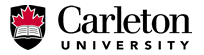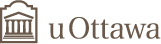# SCIENTIFIC PROGRAMS AND ACTIVITIESJune 26, 2022THE FIELDS INSTITUTE FOR RESEARCH IN MATHEMATICAL SCIENCES
 May 4-5, 2015 Workshop From Lie Algebras To Group Schemes at the University of Ottawa and Carleton University Organizers: • Yuly Billig, Carleton • Erhard Neher, uOttawa • Alistair Savage, uOttawa • Kirill Zaynullin, uOttawa### Abstracts

Yuri Bahturin

One of the uses of affine group schemes is to establish connections between group gradings on finite-dimensional Lie algebras and associative algebras. In this talk I would like to discuss what can be done if the dimension is not necessarily finite.

Howe-Kirillov's orbit method and faithful representation of finite p-groups

A recent result of Karpenko and Merkurjev states that the essential dimension of a p-group G over a field K containing a primitive pth root of unity is equal to the minimal dimension of faithful representations of G over K. Motivated by this result, it is then interesting to compute the minimal dimension of complex faithful representations of a given finite p-group. In this talk I will explain how Lie algebraic method, namely Howe-Kirillov's orbit method, can be applied to answer this question. This is a joint work with Keivan Mallahi-Karai and Hadi Salmasian.

John Binder

Cusp Forms, Fields of Rationality, and Plancherel Equidistribution

I will discuss two questions. First: given a family of (classical) cusp forms, how many of them have Fourier coefficients which generate a "small" number field? Second: given a family of discrete automorphic representations {pi} of a reductive group G, how are the local components {pi_p} distributed? I'll explain the answer to the second question, at least for G = GL_2, and show how this gives an answer to the first question. I'll then discuss what is known (and what is expected!) regarding question 2 for more general groups, and, time permitting explain parts of the proof.

Philippe Gille

Parabolic subgroups of reductive group schemes

We come back to Demazure-Grothendieck's definition of parabolic group schemes for reductive group schemes (SGA3) and will show how this notion is capital for the classification of reductive group schemes over an affine base. At the end we will discuss the special case of Laurent polynomial rings focusing on the work on collaboration with V. Chernousov and A. Pianzola in relation with infinite dimensional Lie theory.

Stefan Gille

Milnor-Witt K-Theory of local rings

Milnor-Witt K-Groups play a prominent role in recent work on the splitting properties of vector bundles over a smooth affine schemes over a field. These groups has been introduced (although not so named) by Barge and Morel some 15 years ago. Morel in collaboration with Hopkins found a presentation of these Milnor-Witt groups of a field of characteristic not 2. In this talk I will present a generalization of this result to local rings which contain an infinite field of characteristic not 2, which has been proven in collaboration with Stephen Scully and Changlong Zhong.

Nikita Karpenko

Incompressibility of products

We show that the conjectural criterion of p-incompressibility for products of projective homogeneous varieties in terms of the factors, previously known in a few special cases only, holds in general. We identify the properties of projective homogeneous varieties actually needed for the proof to go through. For instance, generically split (non-homogeneous) varieties also satisfy these properties.

Mikhail Kochetov

Affine group schemes and duality between gradings and actions

We introduce the concept of affine group scheme and its representing object (a commutative Hopf algebra). Then we explore to what extent the basic results about groups are valid for affine group schemes. In the end, we give one application: an extension of duality between gradings and actions over an algebraically closed field of characteristic zero to the case of an arbitrary field.

Jie Sun

Universal central extensions of twisted current algebras

Twisted current algebras are fixed point subalgebras of tensor products of Lie algebras and associative algebras under finite group actions. Examples of twisted current algebras include equivariant map algebras and twisted forms. In this talk, central extensions of twisted current algebras are constructed and conditions are found under which the construction gives universal central extensions of twisted current algebras.

Qiao Zhou

Affine Grassmannian, Affine Flag Variety, and Their Global Counterparts

I would like to introduce the local and global affine Grassmannian and affine flag variety for a reductive algebraic group \$G\$. Then I will discuss the relations between the geometry of certain objects in the Iwahori orbits in the affine Grassmannian and some representation-theoretic data. Moreover, I will discuss some results related to a degeneration process from a trivial flag variety bundle on the affine Grassmannian to the affine flag variety.

Top